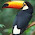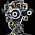4/30/2015

cat detection using latent SVM in opencv

The latent SVM tells the learning method used in this paper -> "Discriminatively trained deformable part models".
The authors site is here. "http://www.cs.berkeley.edu/~rbg/latent/"

This algorithm became known to the researcher as PASCAL challenge won with outstanding ahievement in 2010.

Although the current big trend is deep learning, but we should study the various algorithms.

Matlab source code was opened on the author site.
But the source code is made and tested on the linux and mac os.
If we use the matlab code in the window, it may will need onerous various tasks.

OpenCV provides a detector of latent svm. But learner is not supported.
So we must bring the learning result of matlab.
But I don't know yet how to convert xml file to use openCV function.

Does who know how to convert matlab training result to xml file?

Some researchers have created a c ++ version of the learner of latent svm.

OpenCV introduces 2 version sample code.
"samples/c/latentsvmdetect.cpp" and "samples/cpp/latentsvm_multidetect.cpp"

First code is single object detection example.
Second code is multi class object detection example.

Second code needs one more learned result of objects.
In this article, first code only introduce, because I have only one xml file(cat.xml).

Does anyone have other learned xml result of latent svm ?

I have removed TBB code in origin sample code because some errors.
If you use TBB function, detection processing will be faster.

Detector returns score of detection.
In my case, score is minus.
I don't know yet, the score is right or not.
Now, I am considering more accurate detection value close to zero.
Anyone know?

..
#include <  stdio.h>

#include <  opencv2\opencv.hpp>
#include <  opencv2/highgui/highgui.hpp>
#include <  opencv2\objdetect\objdetect.hpp >

#ifdef _DEBUG
#pragma comment(lib, "opencv_core249d.lib")
#pragma comment(lib, "opencv_objdetect249d.lib")
#pragma comment(lib, "opencv_highgui249d.lib")
#else
#pragma comment(lib, "opencv_core249.lib")
#pragma comment(lib, "opencv_objdetect249.lib")
#pragma comment(lib, "opencv_highgui249.lib")
#endif

using namespace cv;

static void detect_and_draw_objects( IplImage* image, CvLatentSvmDetector* detector);

int main()
{
const char* model_filename = "cat.xml";
const char* image_filename = "cat7.jpg";

IplImage* image = cvLoadImage(image_filename);
CvLatentSvmDetector* detector = cvLoadLatentSvmDetector(model_filename);

detect_and_draw_objects( image, detector );
cvNamedWindow( "test", 1 );
cvShowImage( "test", image );

cvWaitKey(0);
cvReleaseLatentSvmDetector( &detector );
cvReleaseImage( &image );
cvDestroyAllWindows();
}

static void detect_and_draw_objects( IplImage* image, CvLatentSvmDetector* detector)
{

CvMemStorage* storage = cvCreateMemStorage(0);
CvSeq* detections = 0;
int i = 0;
int64 start = 0, finish = 0;

start = cvGetTickCount();
detections = cvLatentSvmDetectObjects(image, detector, storage, 0.5f,100);
finish = cvGetTickCount();
printf("detection time = %.3f\n", (float)(finish - start) / (float)(cvGetTickFrequency() * 1000000.0));

CvFont * font = new CvFont;
cvInitFont(font, CV_FONT_VECTOR0, 0.4f, 0.4f, 0, 1, 8); //rate of width and height is 1:2

for( i = 0; i <  detections->total; i++ )
{
CvObjectDetection detection = *(CvObjectDetection*)cvGetSeqElem( detections, i );
float score         = detection.score;
CvRect bounding_box = detection.rect;
cvRectangle( image, cvPoint(bounding_box.x, bounding_box.y),
cvPoint(bounding_box.x + bounding_box.width,
bounding_box.y + bounding_box.height),
CV_RGB(cvRound(255.0f*score*-1),0,0), 1 );
printf("[%d] - %d %d %d %d : %lf\n", i, bounding_box.x, bounding_box.y, bounding_box.width, bounding_box.height, score);

char szText;
sprintf(szText, "[%d]:%lf", i, score);  //make string
cvPutText(image, szText, cvPoint(bounding_box.x, bounding_box.y+10),
font, CV_RGB(255, 255, 255)); //draw text on the IplImage* (Image)

}
cvReleaseMemStorage( &storage );

}

...

1.1.